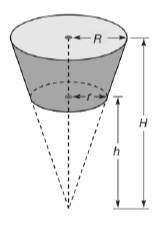Chapter 9.3, Problem 45EElementary Geometry For College St...

7th Edition
Alexander + 2 others
ISBN: 9781337614085

Solutions

Chapter
SectionElementary Geometry For College St...

7th Edition
Alexander + 2 others
ISBN: 9781337614085
Textbook Problem

In Exercises 44 and 45, use the formula from Exercise 43. Similar triangles were used to find h and H.A container of yogurt has the shape of a frustum of a cone. With the lower base having diameter length 6 cm and the upper base having diameter length 8 cm, the volume of such a container 7.5 cm tall can be determined by using H = 30 cm and h = 22.5 cm. Find its volume.A frustum of a cone is the portion of the cone bounded between the circular base and a plane parallel to the base. With dimensions are indicated, show that the volume of the frustum of the cone isV = 1 3 π R 2 H − 1 3 π r h 2

To determine

To find:

The volume of the container.

Explanation

Given:

A container of yogurt has the shape of the frustrum of a cone. With the lower base having diameter 6 cm and the upper base having diameter 8 cm, the volume of such a container 7.5 cm tall can be determined by using H=30 cm, h=22.5 cm.

Formula used:

The volume of the frustum of the cone is V=13πR2H13πr2h.

Calculation:

We have r=3 cm, h=22.5 cm and R=4 cm, H=30 cm

Still sussing out bartleby?

Check out a sample textbook solution.

See a sample solution

The Solution to Your Study Problems

Bartleby provides explanations to thousands of textbook problems written by our experts, many with advanced degrees!

Get Started

In problems 31-38, write the equation of each line described. 34. Through and

Mathematical Applications for the Management, Life, and Social Sciences

93/4

Applied Calculus for the Managerial, Life, and Social Sciences: A Brief Approach

Convert the expressions in Exercises 6584 to power form. 2x3x0.12+43x1.1

Finite Mathematics and Applied Calculus (MindTap Course List)